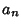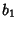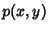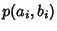## McCoy's Theorem

If two SquareMatrices A and B are simultaneously upper triangularizable by similarity transforms, then there is an ordering, ...,of the Eigenvalues of A and, ...,of the Eigenvalues of B so that, given any Polynomialin noncommuting variables, the Eigenvalues ofare the numberswith, ...,. McCoy's theorem states the converse: If every Polynomial exhibits the correct Eigenvalues in a consistent ordering, then A and B are simultaneously triangularizable.

References

Luchins, E. H. and McLoughlin, M. A. In Memoriam: Olga Taussky-Todd.'' Not. Amer. Math. Soc. 43, 838-847, 1996.